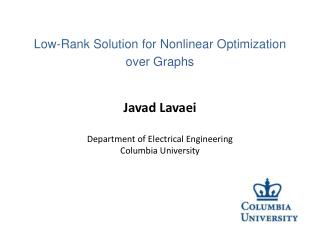Download PresentationJavad Lavaei Department of Electrical Engineering Columbia University

# Javad Lavaei Department of Electrical Engineering Columbia University - PowerPoint PPT Presentation

Javad Lavaei Department of Electrical Engineering Columbia University. Low-Rank Solution for Nonlinear Optimization over Graphs. Acknowledgements. Joint work with Somayeh Sojoudi (Caltech):I am the owner, or an agent authorized to act on behalf of the owner, of the copyrighted work described.
Download Presentation## Javad Lavaei Department of Electrical Engineering Columbia University

Download Policy: Content on the Website is provided to you AS IS for your information and personal use and may not be sold / licensed / shared on other websites without getting consent from its author.While downloading, if for some reason you are not able to download a presentation, the publisher may have deleted the file from their server.

- - - - - - - - - - - - - - - - - - - - - - - - - - E N D - - - - - - - - - - - - - - - - - - - - - - - - - -
Presentation Transcript
1. Javad Lavaei Department of Electrical EngineeringColumbia University Low-Rank Solution for Nonlinear Optimization over Graphs

2. Acknowledgements • Joint work with SomayehSojoudi (Caltech): • S. Sojoudi and J. Lavaei, "Semidefinite Relaxation for Nonlinear Optimization over Graphs," Working draft, 2012. • S. Sojoudi and J. Lavaei, "Convexification of Generalized Network Flow Problem," Working draft, 2012. Javad Lavaei, Columbia University 2

3. Problem of Interest • Abstract optimizations are NP-hard in the worst case. • Real-world optimizations are highly structured: • Sparsity: • Non-trivial structure: • Question: How does the physical structure affect tractability of an optimization? Javad Lavaei, Columbia University 3

4. Example 1 Trick: SDP relaxation: • Guaranteed rank-1 solution! Javad Lavaei, Columbia University 4

5. Example 1 Opt: • Sufficient condition for exactness: Sign definite sets. • What if the condition is not satisfied? • Rank-2 W(but hidden) • NP-hard Javad Lavaei, Columbia University 5

6. Example 2 Opt: Acyclic Graph • Real-valued case: Rank-2 W (need regularization) • Complex-valued case: • Real coefficients: Exact SDP • Imaginary coefficients: Exact SDP • General case: Need sign definite sets Javad Lavaei, Columbia University 6

7. Sign Definite Set • Real-valued case: “T “ is sign definite if its elements are all negative or all positive. • Complex-valued case: “T “ is sign definite if T and –T are separable in R2: Javad Lavaei, Columbia University 7

8. Formal Definition: Optimization over Graph Optimization of interest: (real or complex) Define: • SDP relaxation for y and z (replace xx* with W). • f (y , z) is increasing in z (no convexity assumption). • Generalized weighted graph: weight set for edge (i,j). Javad Lavaei, Columbia University 8

9. Real-Valued Optimization Edge Cycle Javad Lavaei, Columbia University 9

10. Real-Valued Optimization • ExactSDP relaxation: • Acyclic graph: sign definite sets • Bipartite graph: positive weight sets • Arbitrary graph: negative weight sets • Interplay between topology and edge signs Javad Lavaei, Columbia University 10

11. Low-Rank Solution • Violate edge condition: • Satisfy edge condition but violate cycle condition : Javad Lavaei, Columbia University 11

12. Computational Complexity: Acyclic Graph ? • Number partitioning problem: Javad Lavaei, Columbia University 12

13. Complex-Valued Optimization • Main requirement in complex case: Sign definite weight sets • SDP relaxation for acyclic graphs: • real coefficients • 1-2 element sets (power grid: ~10 elements) Javad Lavaei, Columbia University 13

14. Complex-Valued Optimization • Purely imaginary weights (lossless power grid): • Consider a real matrix M: • Polynomial-time solvable for weakly-cyclic bipartite graphs. Javad Lavaei, Columbia University 14

15. Graph Decomposition • There are at least four good structural graphs. • Acyclic combination of them leads to exact SDP relaxation. Opt: • Sufficient conditions for {c12 , c23 ,c13 }: • Real with negative product • Complex with one zero element • Purely imaginary Javad Lavaei, Columbia University 15

16. Resource Allocation: Optimal Power Flow (OPF) Voltage V Current I Complex power = VI*=P + Q i • OPF: Given constant-power loads, find optimal P’s subject to: • Demand constraints • Constraints on V’s, P’s, and Q’s. Javad Lavaei, Columbia University 16

17. Optimal Power Flow Cost Operation Flow Balance • Express the last constraint as an inequality. Javad Lavaei, Columbia University 17

18. Exact Convex Relaxation • OPF: DC or AC • Networks: Distribution or transmission • Result 1: Exact relaxation for DC/AC distribution and DC transmission. • Energy-related optimization: Javad Lavaei, Stanford University Javad Lavaei, Columbia University 17 18

19. Exact Convex Relaxation • Each weight set has about 10 elements. • Due to passivity, they are all in the left-half plane. • Coefficients: Modes of a stable system. • Weight sets are sign definite. Javad Lavaei, Stanford University Javad Lavaei, Columbia University 17 19

20. Generalized Network Flow (GNF) injections flows limits • Goal: • Assumption: • fi(pi): convex and increasing • fij(pij): convex and decreasing Javad Lavaei, Columbia University 20

21. Convexification of GNF Feasible set without box constraint: Monotonic Non-monotonic • Convexification: • It finds correct injection vector but not necessarily correct flow vector. Javad Lavaei, Columbia University 21

22. Convexification of GNF Feasible set without box constraint: • Correct injections in the feasible case. • Why monotonic flow functions? Javad Lavaei, Columbia University 22

23. Conclusions • Motivation: Real-world optimizations are • highly structured. • Goal: Develop theory of optimization over graph • Mapped the structure of an optimization into a generalized weighted graph • Obtained various classes of polynomial-time solvable optimizations • Talked about Generalized Network Flow • Passivity in power systems made optimizations easier Javad Lavaei, Columbia University 23